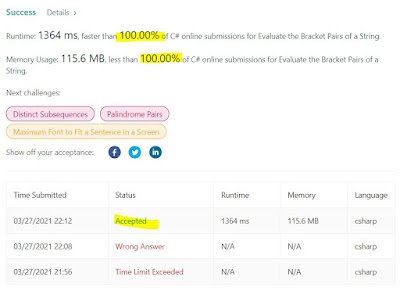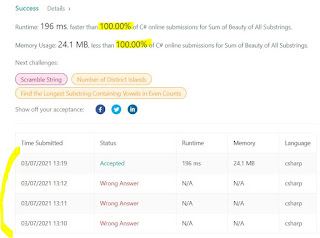## Posts

Showing posts from March, 2021

### Finite-State-Machines (FSM) and avoidance of string manipulation via lazy appendingLatest problem from Leetcode required a combination of FSM and avoidance of string manipulation (in C#, expensive). Here it is:  Evaluate the Bracket Pairs of a String - LeetCode 1807. Evaluate the Bracket Pairs of a String Medium 13 1 Add to List Share You are given a string  s  that contains some bracket pairs, with each pair containing a  non-empty  key. For example, in the string  "(name)is(age)yearsold" , there are  two  bracket pairs that contain the keys  "name"  and  "age" . You know the values of a wide range of keys. This is represented by a 2D string array  knowledge  where each  knowledge[i] = [key i , value i ]  indicates that key  key i  has a value of  value i . You are tasked to evaluate  all  of the bracket pairs. When you evaluate a bracket pair that contains some key  key i , you will: Replace  key i  and the bracket pair with the key's corresponding  value i . If you do not know the value of the key, you will replace  key i  and the

### Trie-Trie-Trie!!!Leetcode is obsessed with Tries. It is indeed a useful data structure, and someone fun to implement with a hashtable and recursion. Here is their latest:  Implement Trie II (Prefix Tree) - LeetCode 1804. Implement Trie II (Prefix Tree) Medium 0 0 Add to List Share A  trie  (pronounced as "try") or  prefix tree  is a tree data structure used to efficiently store and retrieve keys in a dataset of strings. There are various applications of this data structure, such as autocomplete and spellchecker. Implement the Trie class: Trie()  Initializes the trie object. void insert(String word)  Inserts the string  word  into the trie. int countWordsEqualTo(String word)  Returns the number of instances of the string  word  in the trie. int countWordsStartingWith(String prefix)  Returns the number of strings in the trie that have the string  prefix  as a prefix. void erase(String word)  Erases the string  word  from the trie.   Example 1: Input ["Trie", "insert", "

### A brute-force approach for Max BSTI've tried this problem before, twice, with no luck. I remember trying an O(N)-time solution, but got wrong answers twice. Decided to go for an N^2 solution, this time it worked. Here is the problem:  Largest BST Subtree - LeetCode 333. Largest BST Subtree Medium 820 84 Add to List Share Given the root of a binary tree, find the largest subtree, which is also a Binary Search Tree (BST), where the largest means subtree has the largest number of nodes. A  Binary Search Tree (BST)  is a tree in which all the nodes follow the below-mentioned properties: The left subtree values are less than the value of their parent (root) node's value. The right subtree values are greater than the value of their parent (root) node's value. Note:  A subtree must include all of its descendants. Follow up:  Can you figure out ways to solve it with O(n) time complexity?   Example 1: Input: root = [10,5,15,1,8,null,7] Output: 3 Explanation: The Largest BST Subtree in this case is the highlighted

### Center of a Graph, in O(N)-timeProblem is actually not that hard (the acceptance rate is close to 90%), here it is:  Find Center of Star Graph - LeetCode 1791. Find Center of Star Graph Medium 28 45 Add to List Share There is an undirected  star  graph consisting of  n  nodes labeled from  1  to  n . A star graph is a graph where there is one  center  node and  exactly   n - 1  edges that connect the center node with every other node. You are given a 2D integer array  edges  where each  edges[i] = [u i , v i ]  indicates that there is an edge between the nodes  u i  and  v i . Return the center of the given star graph.   Example 1: Input: edges = [[1,2],[2,3],[4,2]] Output: 2 Explanation: As shown in the figure above, node 2 is connected to every other node, so 2 is the center. Example 2: Input: edges = [[1,2],[5,1],[1,3],[1,4]] Output: 1   Constraints: 3 <= n <= 10 5 edges.length == n - 1 edges[i].length == 2 1 <= u i,  v i  <= n u i  != v i The given  edges  represent a valid star graph. Basicall

### Frequency counting for an O(26 * N^2)-timeHere is the problem: https://leetcode.com/problems/sum-of-beauty-of-all-substrings/ 1781. Sum of Beauty of All Substrings Medium 64 42 Add to List Share The  beauty  of a string is the difference in frequencies between the most frequent and least frequent characters. For example, the beauty of  "abaacc"  is  3 - 1 = 2 . Given a string  s , return  the sum of  beauty  of all of its substrings.   Example 1: Input: s = "aabcb" Output: 5 Explanation: The substrings with non-zero beauty are ["aab","aabc","aabcb","abcb","bcb"], each with beauty equal to 1. Example 2: Input: s = "aabcbaa" Output: 17   Constraints: 1 <= s.length <=   500 s  consists of only lowercase English letters. Given relatively small N (500), a solution around N^2 should work. Generating all substrings is an N^2 operation. Keeping the frequency count of all lowercase characters (26) and doing brute-force per substring should be able